# Transimpedance Amplifier - Current to Voltage Converter

Published  July 10, 2019   0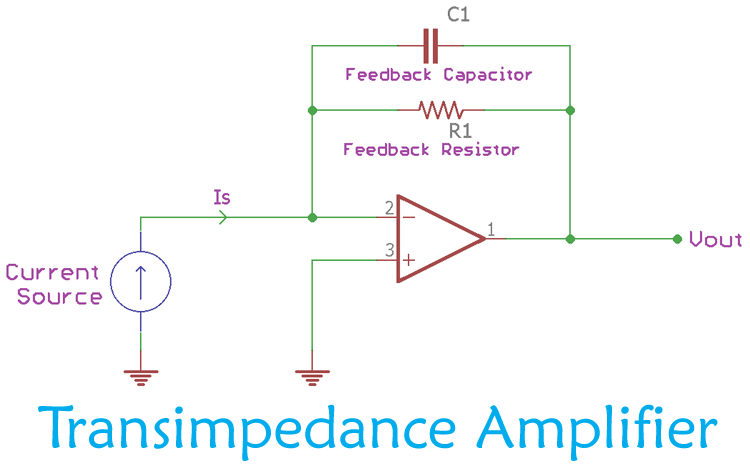To explain in simple words a Transimpedance amplifier is a converter circuit which converts the input current to a proportional output voltage. As we know when current flows through a resistor it creates a voltage drop across the resistor which will be proportional to the value of current and the value resistor itself. Here, assuming the value of resistor to be ideally constant we can easily use Ohms Law to calculate the value of current based on the value of Voltage. This is the most basic Current to Voltage Converter, and since we have used a resistor (Passive element) to accomplish this it is called as a Passive Current to Voltage Converter.

On the other hand a Transimpedance amplifier is an active current to voltage converter since it uses an active component like Op-Amp to convert the input current to a proportional output voltage. It is also possible to build active I to V converters using other active components like  BJTsIGBTsMOSFETs etc. The most commonly used Current to Voltage converter is the Transimpedance Amplifier (TIA), so in this article we will learn more about it and how to use it in your circuit designs.

### Importance of Transimpedance Amplifier

Now that we know even a resistor can be used to convert current to voltage, why do we have to build an active current to voltage converters using Op-Amp? What advantage and importance does it have over Passive V to I converters?

To answer that lets assume a photosensitive diode (current source) is providing current across its terminal depending on the light falling on it and a simple low-value resistor is connected across the photodiode to convert the output current to a proportional voltage as shown in the image below.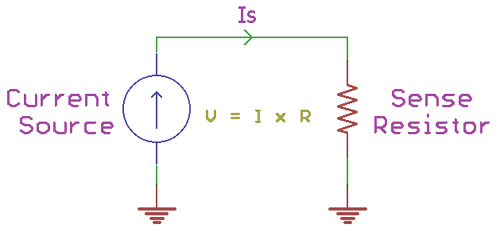The above circuit might work well by theory but in practice the performance will be decorticated because photo-diode will also consist of some unwanted capacitive properties called stray capacitance. Due to this for a smaller value of sense resistor, the time constant (t) (t = sense resistance x Stray Capacitance) will be small and hence the gain will be low. The exact opposite will happen if the sense resistance is increased, the gain will be high and the time constant will also be higher than the small resistor value. This uneven gain will lead to an insufficient signal to noise ratio and the flexibility of the output voltage is limited. Therefore, to fix the poor gain and noise related issues, a Transimpedance amplifier is often preferred. Adding to this in a Transimpedance amplifier, the designer can also configure the bandwidth and the gain response of the circuit as per the design requirements.

### Working of Transimpedance Amplifier

The Transimpedance amplifier circuit is a simple Inverting amplifier with negative feedback. Along with the amplifier, a single feedback resistor (R1) is connected to the inverting end of the Amplifier as shown below.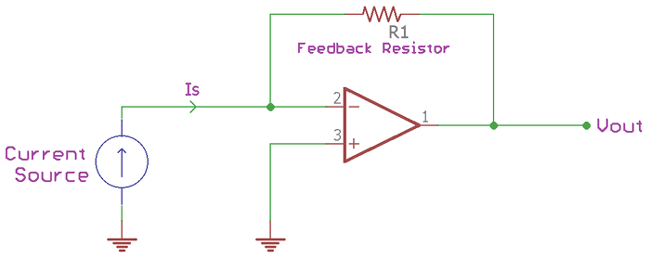As we know the input current of an Op-Amp will be zero due to its high input impedance, hence the current from our current source has to completely pass through resistor R1. Let’s consider this current as Is. At this point, the output voltage (Vout) of the Op-Amp can be calculated using the below formula -

`Vout = -Is x R1`

This formula will hold true in an ideal circuit. But in a real circuit, the op-amp will consist of some value of input capacitance and stray capacitance across its input pins which could cause output drift and ringing oscillation, making the entire circuit unstable. To overcome this problem, instead of a single passive component, two passive components are required for the proper working of the Transimpedance circuit. Those two passive components are the previous resistor (R1) and an additional capacitor (C1). Both the resistor and the capacitor are connected in parallel between the amplifiers negative input and the output as shown below.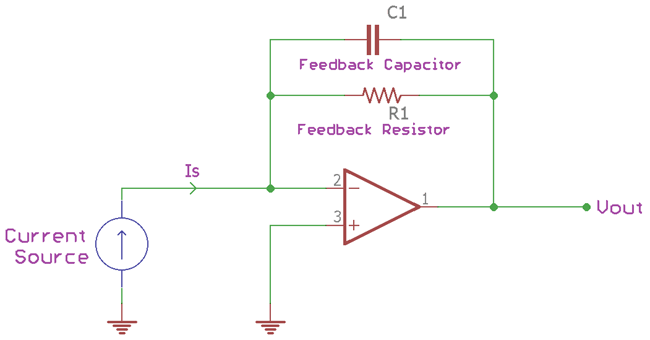The operational amplifier here is again connected in negative feedback condition through the resistor R1 and the capacitor C1 as the feedback. The current (Is) applied to the Inverting pin of the Transimpedance amplifier will be converted into equivalent voltage on the output side as Vout. The value of the input current and the value of resistor (R1) can be used to determine the output voltage of the Transimpedance amplifier.

The output voltage is not only dependent on the feedback resistor, but it is also has a relationship with the value of the feedback capacitor C1. The circuit bandwidth is dependable on the feedback capacitor value C1, therefore this capacitor value can alter the bandwidth of the overall circuit. For the stable operation of the circuit in the entire bandwidth, the formulae to calculate capacitor value for required bandwidth is shown below.

```C1 ≤ 1 / 2π x R1 x fp
```

Where, R1 is the feedback resistor and the fp is the required bandwidth frequency.

In a real situation, parasitic capacitance and the input capacitance of the amplifier plays a vital role in the stability of the Transimpedance amplifier. The noise gain response of the circuit also creates instability due to circuit phase shift margin and cause overshoot step response behavior.

### Transimpedance Amplifier Design

To understand how to use TIA in practical designs let’s design one using a single resistor and capacitor and simulate it to understand its working. The complete circuit for current to voltage converter using Op-amp is shown below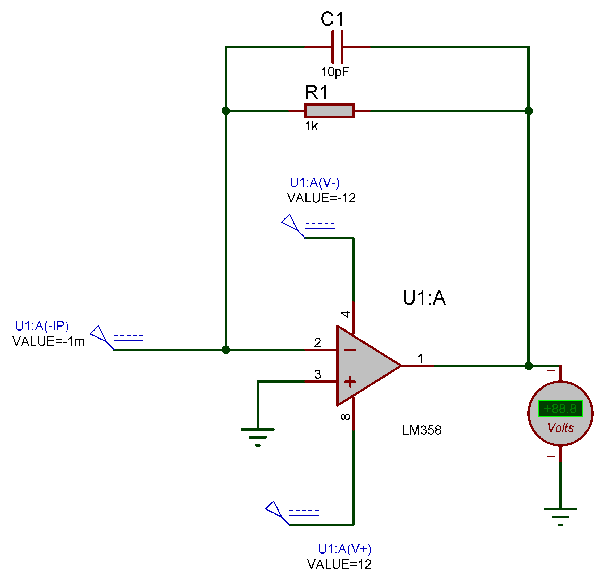The above circuit uses generic low power amplifier LM358. The resistor R1 is acting as a feedback resistor and the capacitor is serving the purpose of a feedback capacitor. The amplifier LM358 is connected in a negative feedback configuration. The negative input pin is connected to a constant current source and the positive pin is connected to the ground or in 0 potential. As it is a simulation and the overall circuit is working closely as an ideal circuit the capacitor value wouldn’t affect much but it is essential if the circuit is constructed physically. 10pF is a reasonable value but the capacitor value can be changed depending on the frequency bandwidth of the circuitry which can be calculated using C1 ≤ 1 / 2π x R1 x fp as discussed earlier.

For the perfect operation, the op-amp also gets power from a dual power rail supply which is +/- 12V. The feedback resistor value is selected as 1k.

### Transimpedance amplifier Simulation

The above circuit can be simulated to check if the design works as expected. A DC voltmeter is connected across the op-amp output to measure the output voltage of our Transimpedance amplifier. If the circuit is working properly, then the value of output voltage displayed on the voltmeter should be proportional to the current applied to the inverting pin of the Op-Amp.

The complete simulation video can be found below

In the test case 1, the input current across the op-amp is given as 1mA. As the input impedance of the op-amp is very high, the current start to flow through the feedback resistor and the output voltage is dependable on the feedback resistor value times the current is flowing, governed by the formula Vout = -Is x R1 as we discussed earlier.

In our circuit the value of Resistor R1 is 1k. Therefore, when the input current is 1mA, the Vout will be,

```Vout = -Is x R1
Vout = -0.001 Amp x 1000 Ohms [1mA and the 1 Kilo-Ohms]
Vout = 1 Volt```

If we check our Current to Voltage simulation result, it matches exactly. The output became positive by the effect of Transimpedance amplifier.In the test case 2, the input current across the op-amp is given as .05mA or 500 microamperes. Therefore the value of output voltage can be calculated as.

```Vout = -Is x R1
Vout = -0.0005 Amp x 1000 Ohms [1mA and the 1 Kilo-Ohms]
Vout = .5 Volt```

If we check the simulation result, this also matches exactly.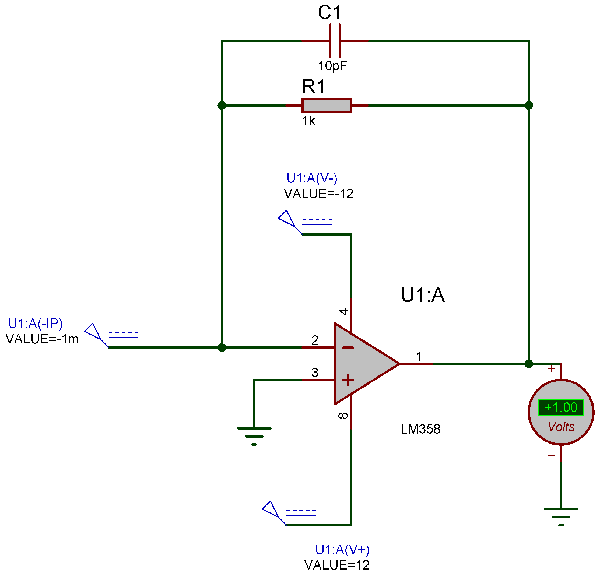Once again this is a simulation result. While building the circuit practically simple stray capacitance could produce time constant effect in this circuit. The designer should be careful about the below points when constructing physically.

1. Avoid breadboards or copper clad boards or any other strip boards for connection. Build the circuit only on PCB.
2. The Op-Amp need to be soldered directly to the PCB without IC holder.
3. Use short traces for feedback paths and the input current source (Photodiode or similar things that are needed to be measured by a Transimpedance amplifier).
4. Place the feedback resistor and the capacitor as close as possible to the operational amplifier.
5. It is good to use short leaded resistors.
6. Add proper filter capacitors with both large and small values on the power supply rail.
7. Choose proper op-amp specially designed for this purpose of the amplifier for simplicity of the design.

### Applications of Transimpedance Amplifier

A Transimpedance amplifier is the most essential current signal measurement tool for light sensing related operation. It is widely used in chemical engineering, pressure transducers, different types of accelerometers, advanced driver assistance systems and LiDAR technology which is used in autonomous vehicles.

The most critical part of the Transimpedance circuit is design stability. This is because of the parasitic and the noise related issues. The designer must be careful about choosing the right amplifier and should be careful to use proper PCB guidelines.